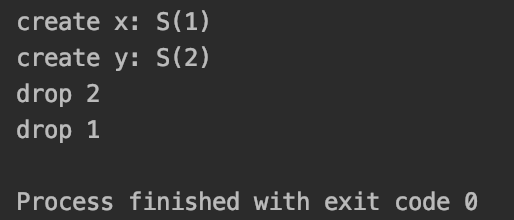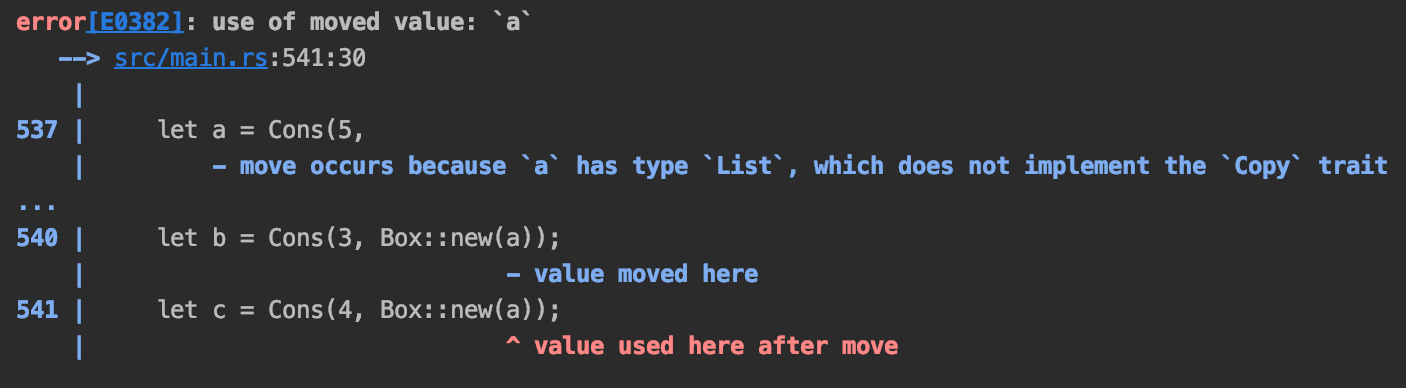# Rust入坑指南：智能指针

2019/04/10 10:10

### 基本概念

#### Deref

Deref有一个特性是强制隐式转换：如果一个类型T实现了Deref<Target=U>，则该类型T的引用在应用的时候会被自动转换为类型U

``````use std::rc::Rc;
fn main() {
let x = Rc::new("hello");
println!("{:?}", x.chars());
}
``````

``````#[stable(feature = "rust1", since = "1.0.0")]
impl<T: ?Sized> Deref for Rc<T> {
type Target = T;

#[inline(always)]
fn deref(&self) -> &T {
&self.inner().value
}
}
``````

Deref的内部实现是这样的：

``````#[lang = "deref"]
#[doc(alias = "*")]
#[doc(alias = "&*")]
#[stable(feature = "rust1", since = "1.0.0")]
pub trait Deref {
/// The resulting type after dereferencing.
#[stable(feature = "rust1", since = "1.0.0")]
type Target: ?Sized;

/// Dereferences the value.
#[must_use]
#[stable(feature = "rust1", since = "1.0.0")]
fn deref(&self) -> &Self::Target;
}

#[lang = "deref_mut"]
#[doc(alias = "*")]
#[stable(feature = "rust1", since = "1.0.0")]
pub trait DerefMut: Deref {
/// Mutably dereferences the value.
#[stable(feature = "rust1", since = "1.0.0")]
fn deref_mut(&mut self) -> &mut Self::Target;
}
``````

DerefMut和Deref类似，只不过它是返回可变引用的。

#### Drop

Drop对于智能指针非常重要，它是在智能指针被丢弃时自动执行一些清理工作，这里所说的清理工作并不仅限于释放堆内存，还包括一些释放文件和网络连接等工作。之前我总是把Drop理解成Java中的GC，随着对它的深入了解后，我发现它比GC要强大许多。

Drop的内部实现是这样的：

``````#[lang = "drop"]
#[stable(feature = "rust1", since = "1.0.0")]
pub trait Drop {
#[stable(feature = "rust1", since = "1.0.0")]
fn drop(&mut self);
}
``````

``````use std::ops::Drop;
#[derive(Debug)]
struct S(i32);

impl Drop for S {
fn drop(&mut self) {
println!("drop {}", self.0);
}
}

fn main() {
let x = S(1);
println!("create x: {:?}", x);
{
let y = S(2);
println!("create y: {:?}", y);
}
}
``````### Box<T>

``````fn main() {
let x = Box::new("hello");
println!("{:?}", x.chars())
}
``````

``````#[stable(feature = "rust1", since = "1.0.0")]
#[inline(always)]
pub fn new(x: T) -> Box<T> {
box x
}
``````

``````#[cfg(not(test))]
#[lang = "exchange_malloc"]
#[inline]
unsafe fn exchange_malloc(size: usize, align: usize) -> *mut u8 {
if size == 0 {
align as *mut u8
} else {
let layout = Layout::from_size_align_unchecked(size, align);
let ptr = alloc(layout);
if !ptr.is_null() {
ptr
} else {
handle_alloc_error(layout)
}
}
}

#[cfg_attr(not(test), lang = "box_free")]
#[inline]
pub(crate) unsafe fn box_free<T: ?Sized>(ptr: Unique<T>) {
let ptr = ptr.as_ptr();
let size = size_of_val(&*ptr);
let align = min_align_of_val(&*ptr);
// We do not allocate for Box<T> when T is ZST, so deallocation is also not necessary.
if size != 0 {
let layout = Layout::from_size_align_unchecked(size, align);
dealloc(ptr as *mut u8, layout);
}
}
``````

### Rc<T>

Rc<T>是单线程引用计数指针，不是线程安全类型。``````enum List {
Cons(i32, Box<List>),
Nil,
}

use crate::List::{Cons, Nil};

fn main() {
let a = Cons(5,
Box::new(Cons(10,
Box::new(Nil))));
let b = Cons(3, Box::new(a));
let c = Cons(4, Box::new(a));
}
````````````enum List {
Cons(i32, Rc<List>),
Nil,
}

use crate::List::{Cons, Nil};
use std::rc::Rc;

fn main() {
let a = Rc::new(Cons(5, Rc::new(Cons(10, Rc::new(Nil)))));
let b = Cons(3, Rc::clone(&a));
let c = Cons(4, Rc::clone(&a));
println!("count a {}", Rc::strong_count(&a));
}
``````

Rust还为我们提供了另一种智能指针Weak<T>，你可以把它当作是Rc<T>的另一个版本。它提供的引用属于弱引用。它共享的指针没有所有权。但他可以帮助我们有效的避免循环引用。

### RefCell<T>

``````struct User {
id: i32,
name: str,
age: u8,
}
``````

``````use std::cell::Cell;
struct Foo {
x: u32,
y: Cell<u32>,
}

fn main() {
let foo = Foo { x: 1, y: Cell::new(3)};
assert_eq!(1, foo.x);
assert_eq!(3, foo.y.get());
foo.y.set(5);
assert_eq!(5, foo.y.get());
}
``````

``````use std::cell::RefCell;
fn main() {
let x = RefCell::new(vec![1, 2, 3]);
println!("{:?}", x.borrow());
x.borrow_mut().push(5);
println!("{:?}", x.borrow());
}
``````

`RefCell<T>``Cell<T>`还有一点区别是：`Cell<T>`没有运行时开销（不过也不要用它包裹大的数据结构），而`RefCell<T>`是有运行时开销的，这是因为使用`RefCell<T>`时需要维护一个借用检查器，如果违反借用规则，则会引起线程恐慌。

0
0 收藏

### 作者的其它热门文章0 评论
0 收藏
0